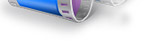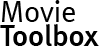﻿ MovieToolbox Audio/Video encyclopedia:: Bit rate

Contacts | Site MapProducts

Freeware\$39,00
for one price

# Bit rate

In telecommunications and computing, bitrate (sometimes written bit rate, data rate or as a variable R or fb) is the number of bits that are conveyed or processed per unit of time.
The bit rate is quantified using the bits per second (bit/s or bps) unit, often in conjunction with an SI prefix such as kilo- (kbit/s or kbps), mega- (Mbit/s or Mbps), giga- (Gbit/s or Gbps) or tera- (Tbit/s or Tbps). 1 kbit/s has almost always meant 1,000 bit/s, not 1,024 bit/s, also before 1999 when SI prefixes were defined for units of information in an IEC standard.
The formal abbreviation for "bits per second" is "bit/s" (not "bits/s"). In less formal contexts the abbreviations "b/s" or "bps" are often used, though this risks confusion with "bytes per second" ("B/s", "Bps").
Multimedia bit rate
In digital multimedia, bit rate often refers to the number of bits used per unit of playback time to represent a continuous medium such as audio or video after source coding (data compression). The size of a multimedia file in bytes is the product of the bit rate (in bit/s) and the length of the recording (in seconds), divided by eight.
In case of streaming multimedia, this bit rate measure is the goodput that is required to avoid interrupts. For streaming multimedia without interrupts, we have the following relationship: Multimedia bit rate = Required goodput The term average bitrate (ABR) is used in case of variable bitrate multimedia source coding schemes.
A theoretical lower bound for the multimedia bit rate for lossless data compression is the source information rate, also known as the entropy rate.
The article is based on materials from matroska.org, wikipedia.org.
Video Joiner Video Converter DVD Ripper MPEG Converter AVI Joiner